### Geometry II

Now let's extend our knowledge of Thales' theorem to a new case.

How are the values of $x$ and $y$ related in the figure below?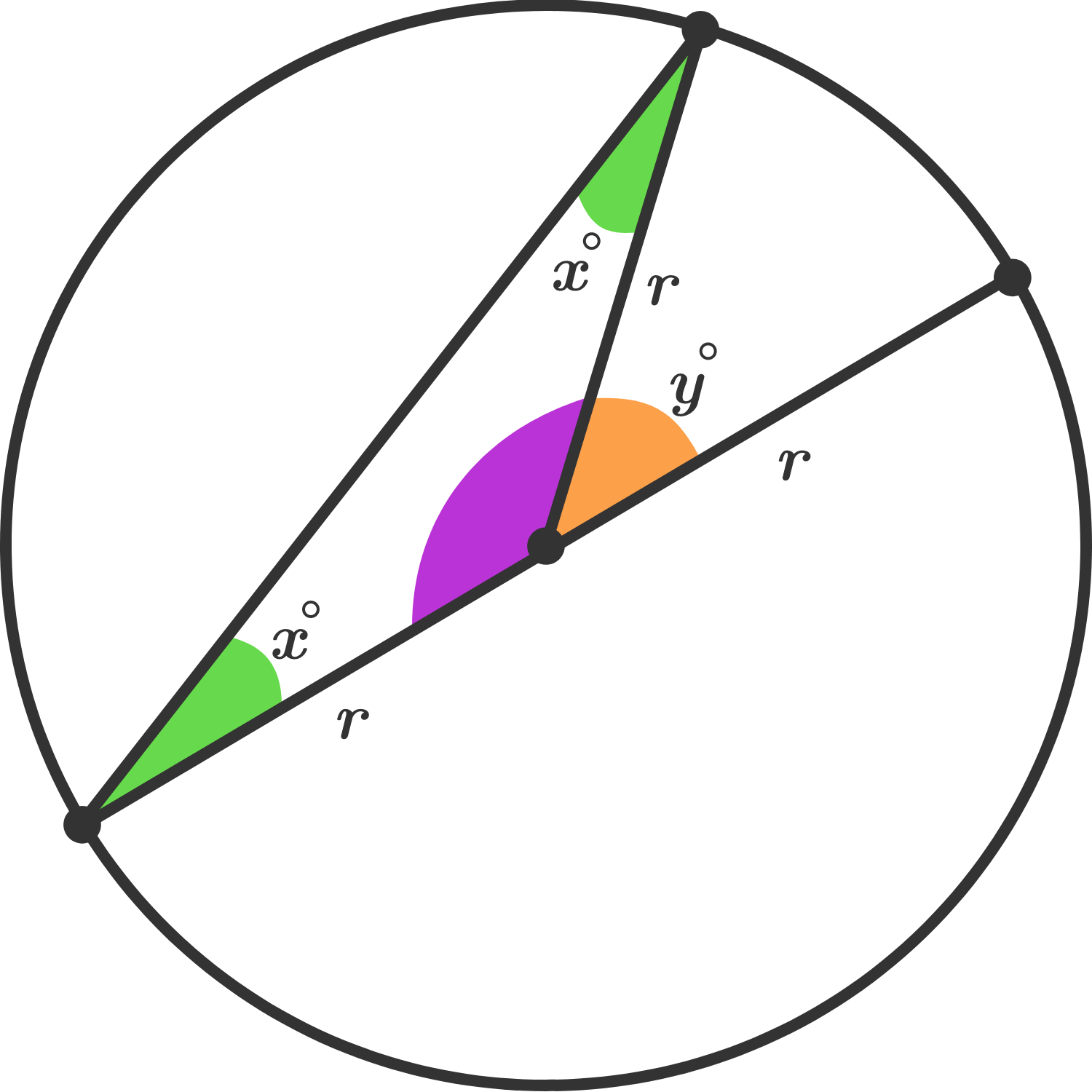The green angles are inscribed angles and the orange angle is a central angle.

We can see that $y$ is larger than $x,$ but we want to determine how much larger. If we know that the purple angle measures $180^\circ - y,$ then what can we say about how $x$ and $y$ compare?

# Inscribed Angles

Using the diagram below and our previous theorems, we know that $b = 2a$ and that $y = 2x.$

Is it always, sometimes, or never true that $b+y = 2(a+x) ?$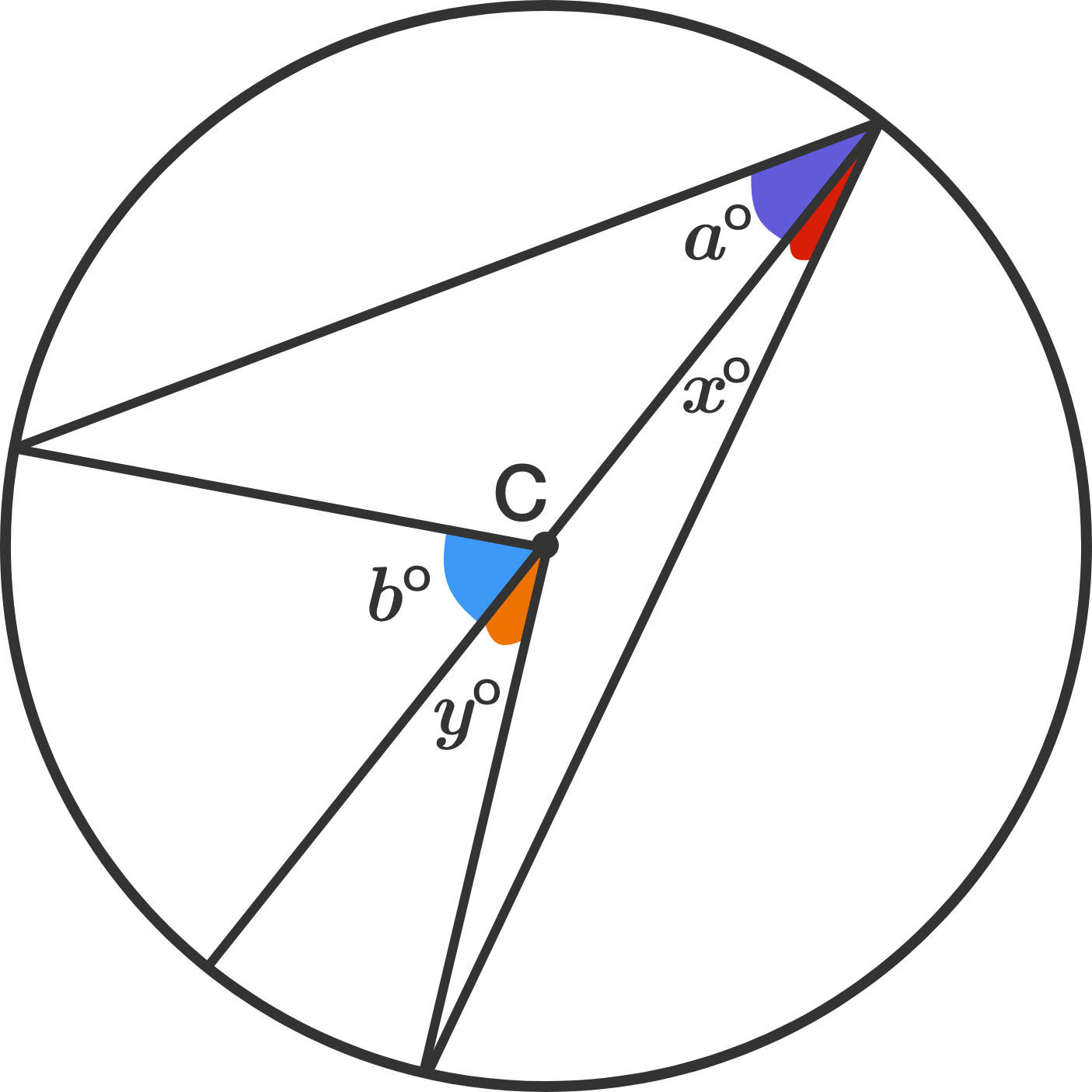# Inscribed Angles

Congratulations! You've now proven the inscribed angles theorem!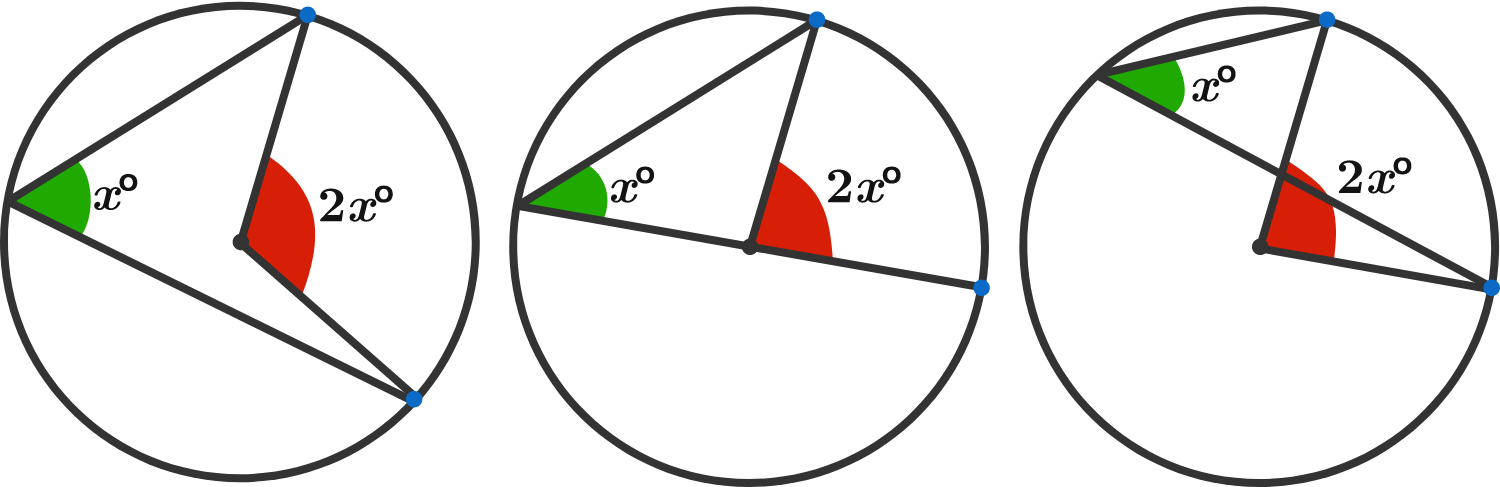As we saw in the last few problems, central angles and inscribed angles have a special relationship:

Whenever a central angle and an inscribed angle share the same endpoints, the measure of the central angle will always be twice the measure of the inscribed angle.

This property is known as the inscribed angle theorem, and over the last several problems, you proved it!

The image above illustrates three cases that look different, but all three are instances when the inscribed angles theorem would apply.

A quick reminder of the relevant definitions:

An inscribed angle is an angle on the interior of a circle that forms where two chords meet: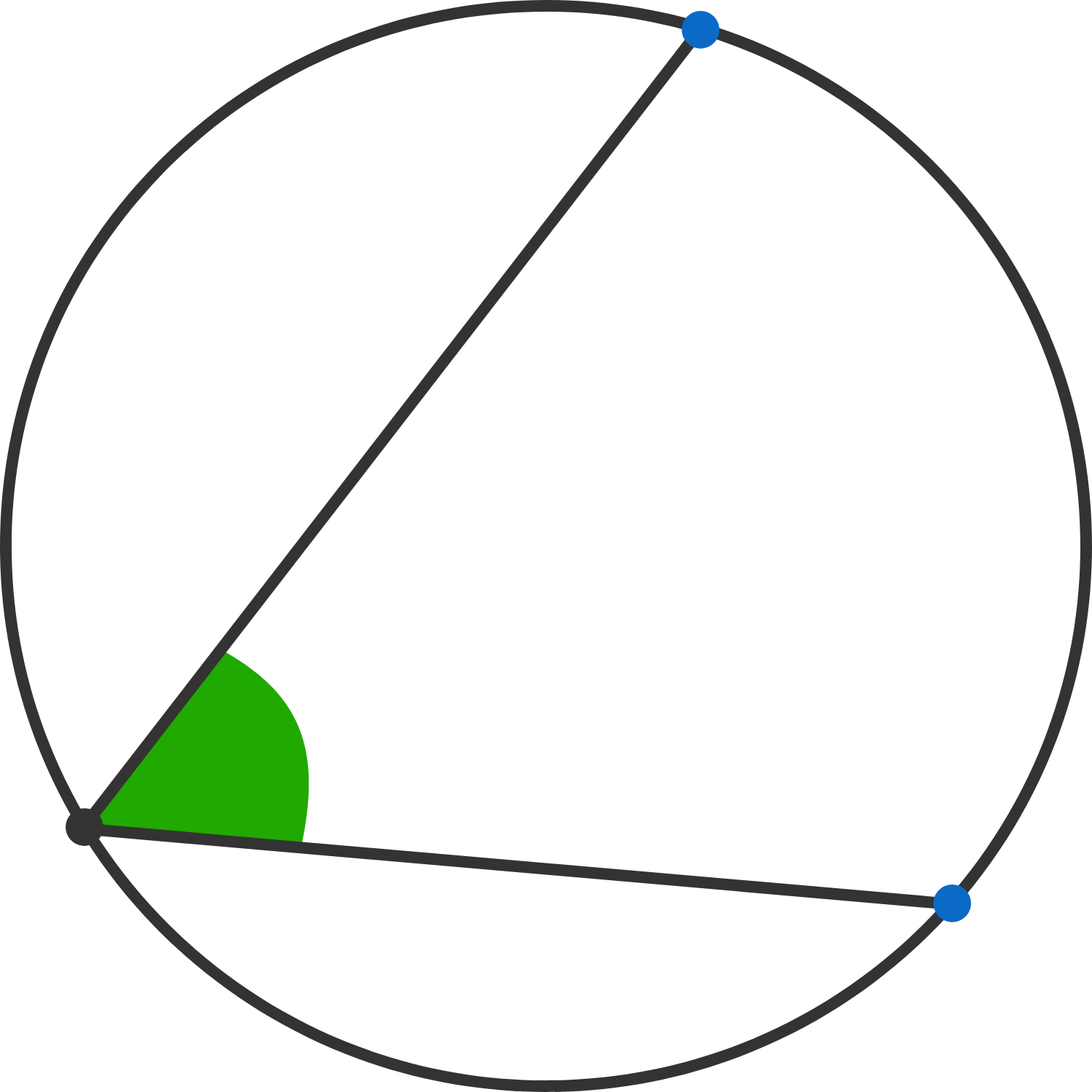A central angle is an angle on the interior of a circle whose vertex is the center of the circle and whose legs (sides) are radii: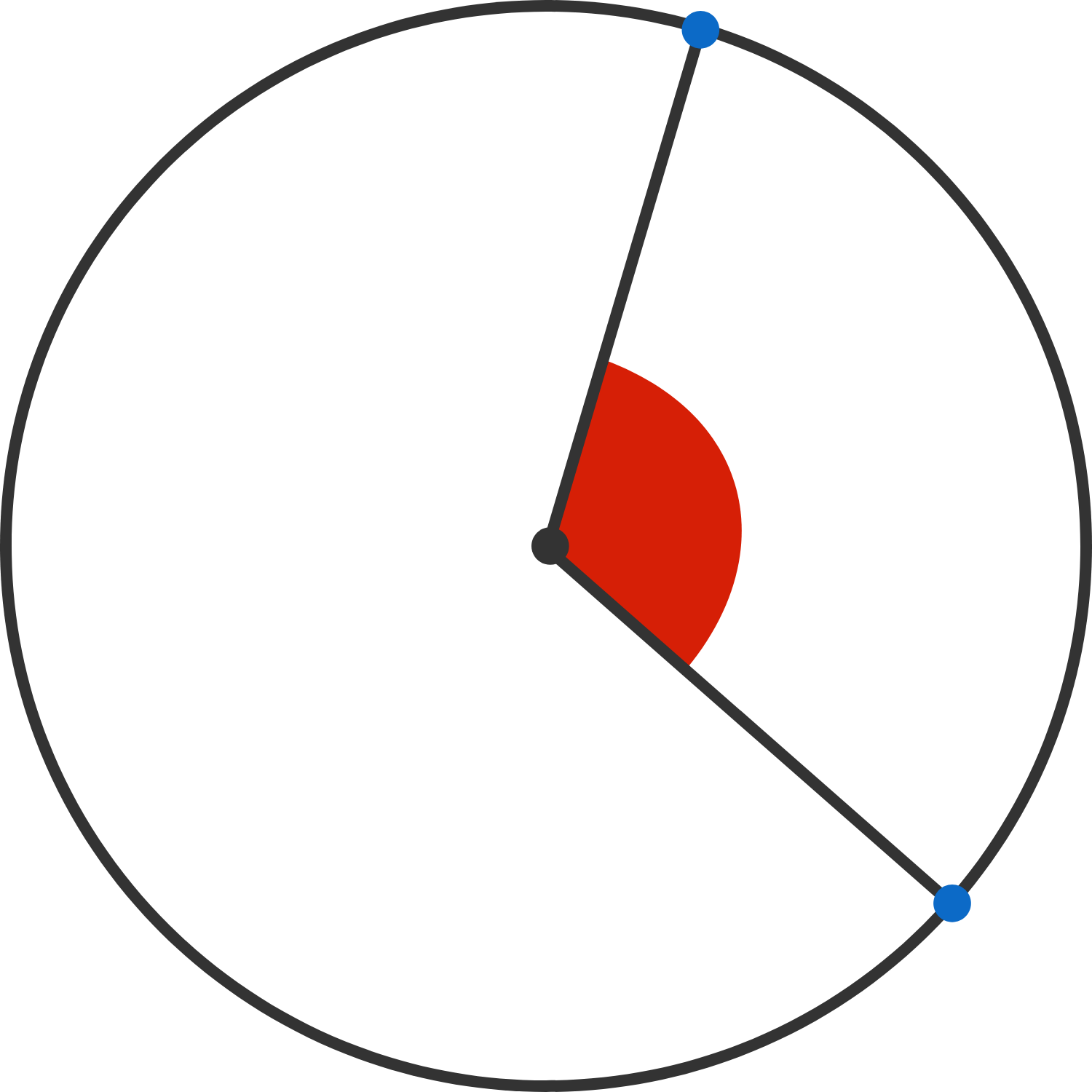# Inscribed Angles

Applying the Inscribed Angle Theorem:

True or False?

From the diagram below, we can definitely conclude $x = y .$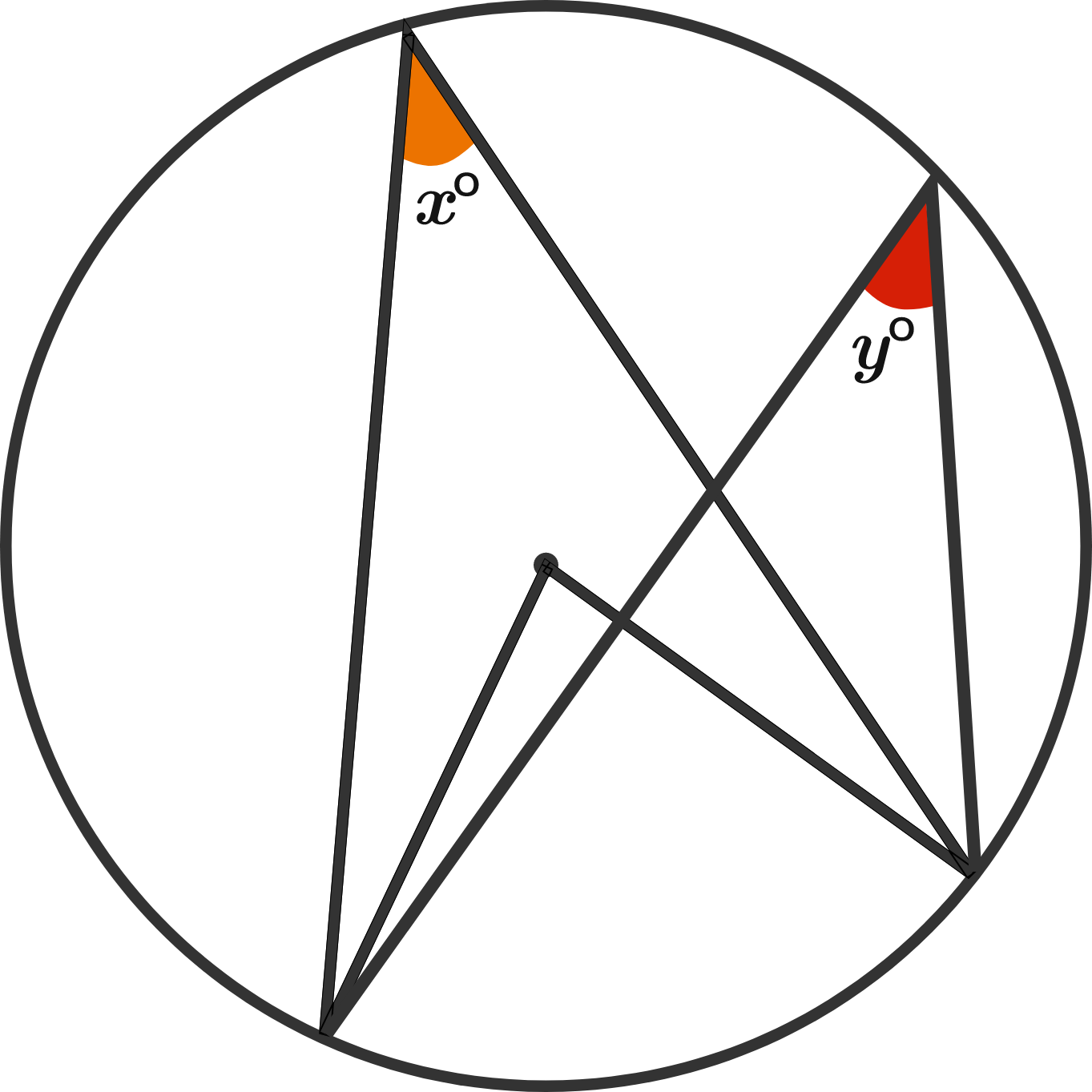# Inscribed Angles

What is the value of $x\,?$ The orange angle has its vertex at the center of the circle.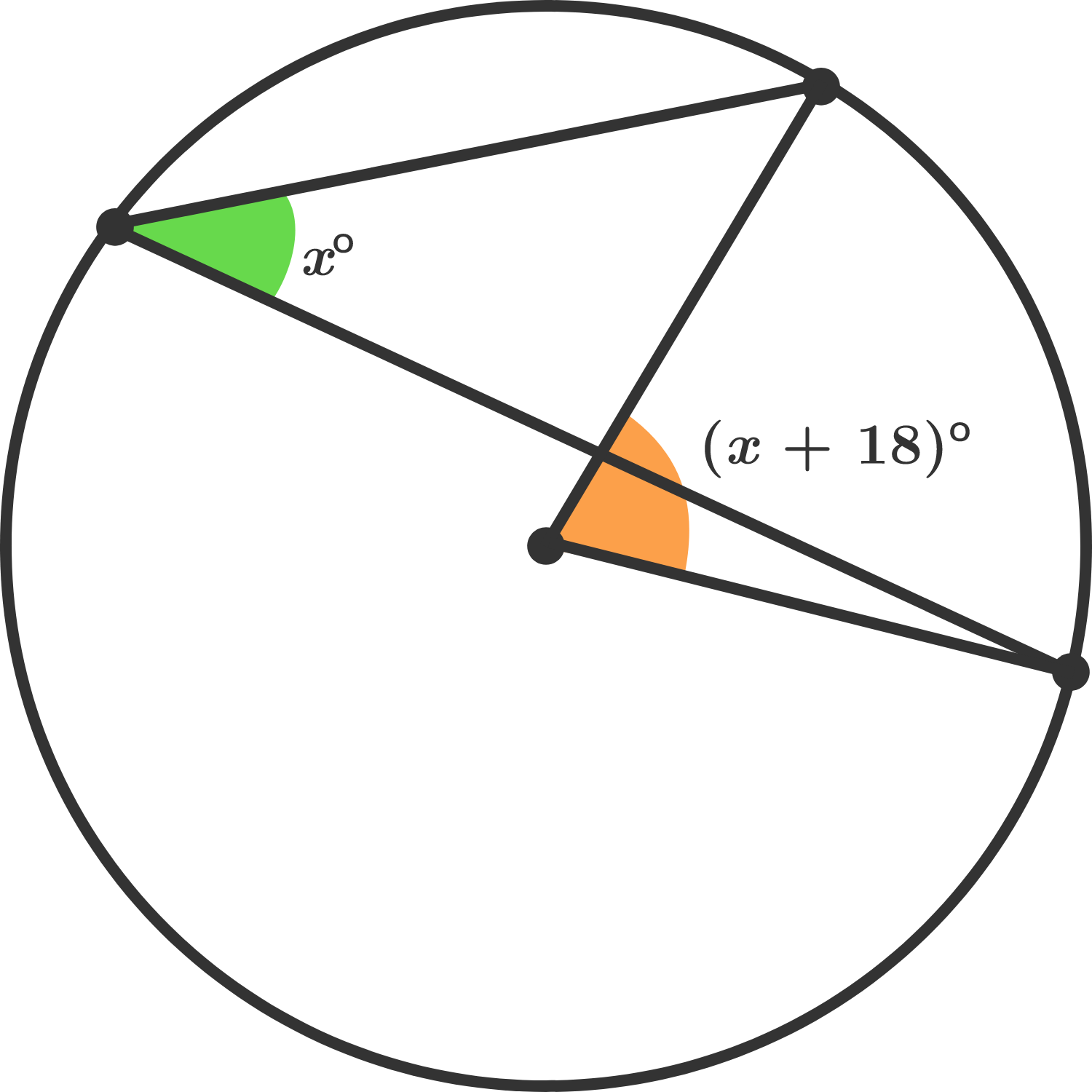# Inscribed Angles

Applying the Inscribed Angle Theorem:

What is the value of $r + b\,?$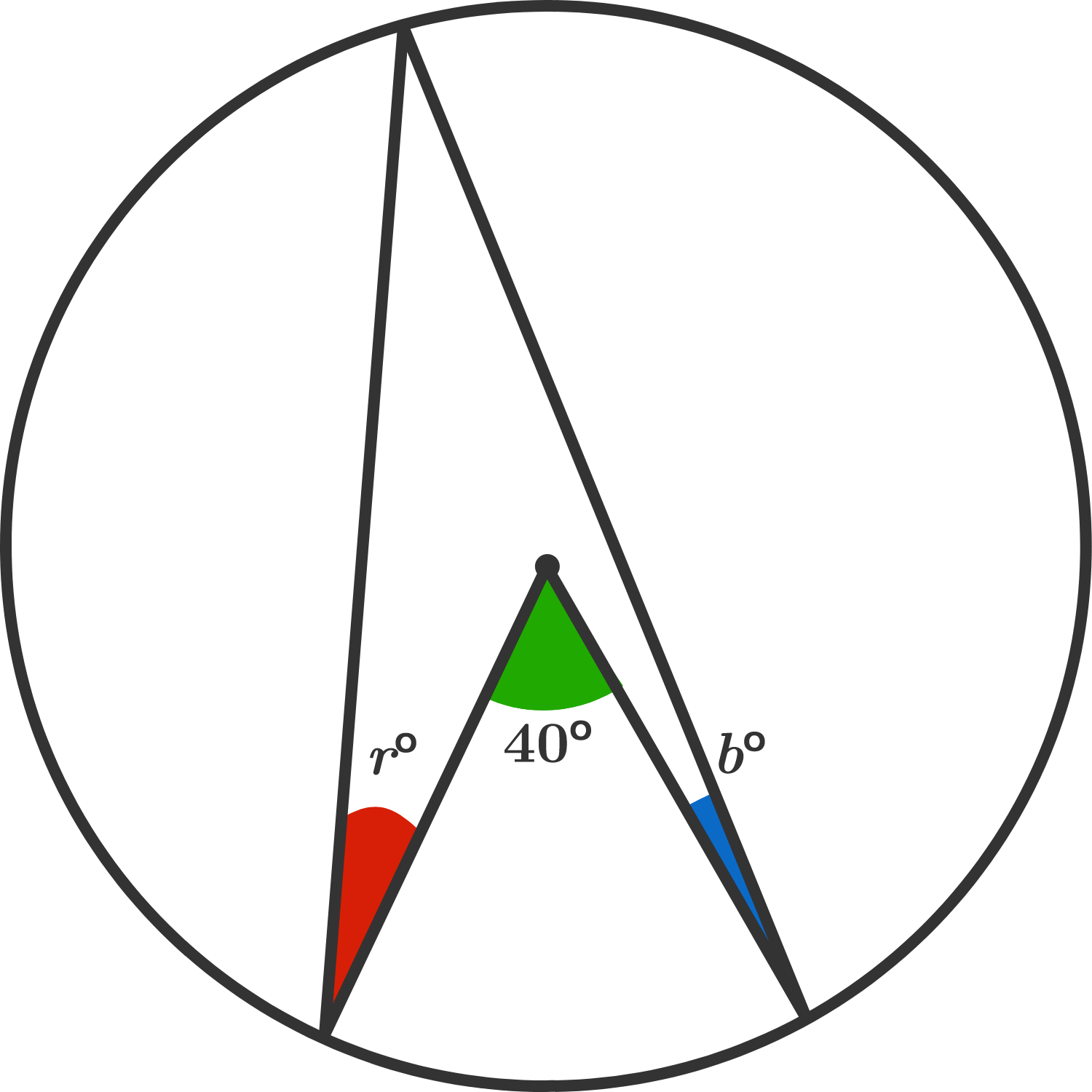# Inscribed Angles

So far, you've learned about angles in circles, Thales' Theorem, and the Inscribed Angle Theorem.In the main course, you'll take on case #3 of the Inscribed Angle Theorem and then discover many more geometric theorems, including one of the most astonishing of all, Euler's line.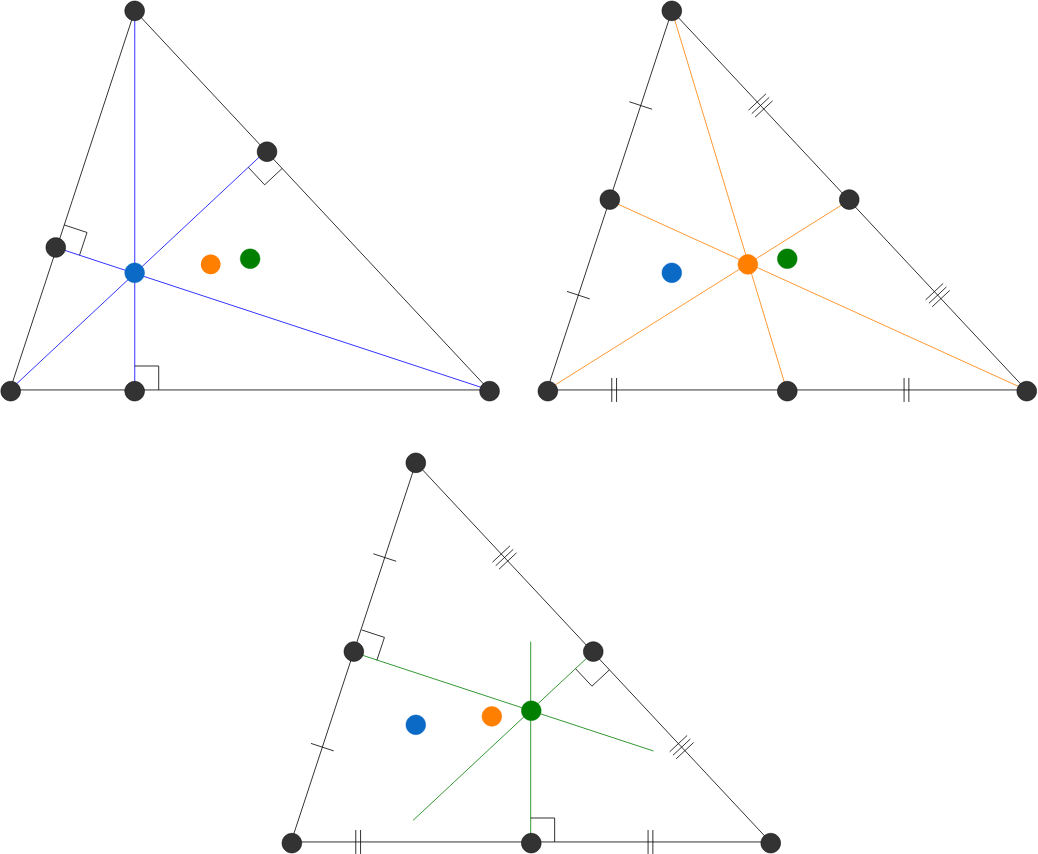×